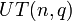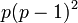# Element structure of unitriangular matrix group:UT(3,p)

This article gives specific information, namely, element structure, about a family of groups, namely: unitriangular matrix group:UT(3,p).
View element structure of group families | View other specific information about unitriangular matrix group:UT(3,p)

This article describes in detail the element structure of the unitriangular matrix group:UT(3,p) for a prime number$p$. This is a special case of unitriangular matrix group of degree three over a finite field. For the element structure of this larger family, see element structure of unitriangular matrix group of degree three over a finite field.

The case$p = 2$, which gives dihedral group:D8, behaves somewhat differently from the case$p$ odd.

## Summary

Item Value
number of conjugacy classes$p^2 + p - 1$
order$p^3$
Agrees with general order formula for$UT(n,q)$:$q^{n(n-1)/2} = p^{(3)(2)/2} = p^3$
conjugacy class size statistics size 1 ($p$ times), size$p$ ($p^2 - 1$ times)
orbits under automorphism group Case$p = 2$: size 1 (1 conjugacy class of size 1), size 1 (1 conjugacy class of size 1), size 2 (1 conjugacy class of size 2), size 4 (2 conjugacy classes of size 2 each)
Case odd$p$: size 1 (1 conjugacy class of size 1), size$p - 1$ ($p - 1$ conjugacy classes of size 1 each), size$p^3 - p$ ($p^2 - 1$ conjugacy classes of size$p$ each)
number of orbits under automorphism group 4 if$p = 2$
3 if$p$ is odd
order statistics Case$p = 2$: order 1 (1 element), order 2 (5 elements), order 4 (2 elements)
Case$p$ odd: order 1 (1 element), order$p$ ($p^3 - 1$ elements)
exponent 4 if$p = 2$$p$ if$p$ odd

## Conjugacy class structure

### Number of conjugacy classes

The general theory says that number of conjugacy classes in unitriangular matrix group of fixed degree over a finite field is polynomial function of field size, where the degree of the polynomial is one less than the degree of matrices. Thus, we expect that the number of conjugacy classes is a polynomial function of the field size$q$ (which here equals the prime$p$) of degree 3 - 1 = 2. Indeed, this is the case, and the explicit polynomial is$p^2 + p - 1$.

### Conjugacy class structure in the unitriangular matrix group

Note that the characteristic polynomial of all elements in this group is$(t - 1)^3$, hence we do not devote a column to the characteristic polynomial.

For reference, we consider matrices of the form:$\begin{pmatrix} 1 & a_{12} & a_{13} \\ 0 & 1 & a_{23} \\ 0 & 0 & 1 \\\end{pmatrix}$

Nature of conjugacy class Jordan block size decomposition Minimal polynomial Size of conjugacy class Number of such conjugacy classes Total number of elements Order of elements in each such conjugacy class Type of matrix
identity element 1 + 1 + 1 + 1$t - 1$ 1 1 1 1$a_{12} = a_{13} = a_{23} = 0$
non-identity element, but central (has Jordan blocks of size one and two respectively) 2 + 1$(t - 1)^2$ 1$p - 1$$p - 1$$p$$a_{12} = a_{23} = 0$,$a_{13} \ne 0$
non-central, has Jordan blocks of size one and two respectively 2 + 1$(t - 1)^2$$p$$2(p - 1)$$2p(p - 1)$$p$$a_{12}a_{23} = 0$, but not both$a_{12}$ and$a_{23}$ are zero
non-central, has Jordan block of size three 3$(t - 1)^3$$p$$(p - 1)^2$$p(p - 1)^2$$p$ if$p$ odd
4 if$p = 2$
both$a_{12}$ and$a_{23}$ are nonzero
Total (--) -- -- --$p^2 + p - 1$$p^3$ -- --

### Grouping by conjugacy class sizes

Conjugacy class size Total number of conjugacy classes of this size Total number of elements Cumulative number of conjugacy classes Cumulative number of elements
1$p$$p$$p$$p$$p$$p^2 - 1$$p^3 - p$$p^2 + p - 1$ (total)$p^3$ (total)

### Conjugacy classes with respect to the general linear group

If we consider the action of the general linear group by conjugation, then there is considerable fusion of conjugacy classes. Specifically, there are only three equivalence classes, corresponding to the set of unordered integer partitions of 3 describing the possible Jordan block decompositions.

Below is a summary of the information:

Jordan block size decomposition (partition of 3) Number of conjugacy classes of size 1
Number of elements in these
Number of conjugacy classes of size$q$
Number of elements in these
Total (number of conjugacy classes, elements)
1 + 1 + 1 1
1
0
0
1
1
2 + 1$p - 1$$p - 1$$2(p - 1)$$2p(p - 1)$$3(p - 1)$$(p - 1)(2p + 1)$
3 0
0$(p - 1)^2$$p(p - 1)^2$$(p - 1)^2$$p(p - 1)^2$
Total (--)$p$$p$$p^2 - 1$$p^3 - p$$p^2 + p - 1$$p^3$